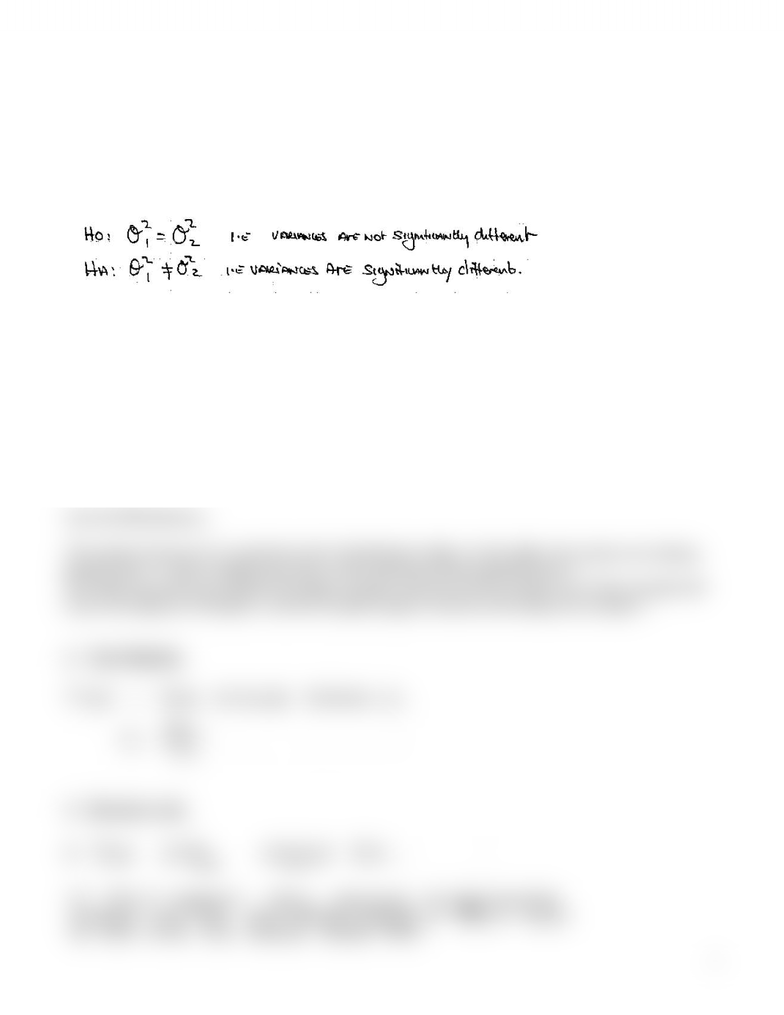# Variance Ratio Test

42 views1 pages
School
Department
Course
Professor1
Variance Ratio Test
¾ Used to test whether the population (and sample) variances are equal.
¾ The answer to this question will determine which version of the t statistic in the two sample
means test is used.
¾ The test uses the F distribution, which is skewed.
1. Hypotheses
*HQHUDOIRUP«
H0: No significant difference between the variance of sample one and the variance of sample 2.
Therefore, the samples are drawn from the same population.
HA: There is a significant difference significant difference between the variance of sample 1 and
the variance of sample 2. Therefore, the samples are drawn from different populations.
2. Critical Value
Use the Fdistribution,
The critical value for F is read from the F distribution table. In this table, the values are always
greater than 1, which implies the ratio of the variances will be greater than 1.
The table assumes you divide the larger sample variance by the smaller one. Each sample will
have one degree of freedom, and the sample largest variance will always be sample 1.
3. Test Statistic
4. Decision rule.
www.notesolution.com
Unlock document

This preview shows half of the first page of the document.
Unlock all 1 pages and 3 million more documents.

## Document Summary

Used to test whether the population (and sample) variances are equal. The answer to this question will determine which version of the t statistic in the two sample. The test uses the f distribution, which is skewed: hypotheses. H0: no significant difference between the variance of sample one and the variance of sample 2. Therefore, the samples are drawn from the same population. Ha: there is a significant difference significant difference between the variance of sample 1 and the variance of sample 2. Therefore, the samples are drawn from different populations: critical value. The critical value for f is read from the f distribution table. In this table, the values are always greater than 1, which implies the ratio of the variances will be greater than 1. The table assumes you divide the larger sample variance by the smaller one.

## Get access